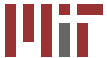Department of Electrical Engineering and Computer Science  Department of Mechanical Engineering 6.050J / 2.110J     Information and Entropy     Spring 2003

## Notes

These notes are for Spring 2003.

Note that these notes are still under development. We would appreciate learning of any errors, and would be pleased to receive suggestions for improvements. Please use the comment form linked below.

Preface (version 1.0.2)

Chapter 1. Bits (version 1.0.2)

Chapter 2. Codes (version 1.0.2)

Chapter 3. Compression (version 1.0.2)

Chapter 4. Errors (version 1.0.2)

Chapter 5. Probability (version 1.0.2)

Chapter 6. Communications (version 1.0.2)

Chapter 7. Processes (version 1.0.2)

Chapter 8. Inference (version 1.0.2)

Chapter 9. Principle of Maximum Entropy: Simple Form (version 1.0.2)

Chapter 10. Principle of Maximum Entropy (version 1.0.2)

Chapter 11. Physical Systems (version 1.0.2)

Chapter 12. Energy (version 1.0.2)

Chapter 13. Temperature (version 1.0.2)

Chapter 14. Quantum Information (version 1.0.2)